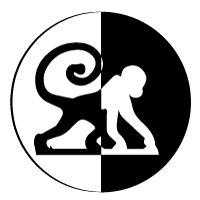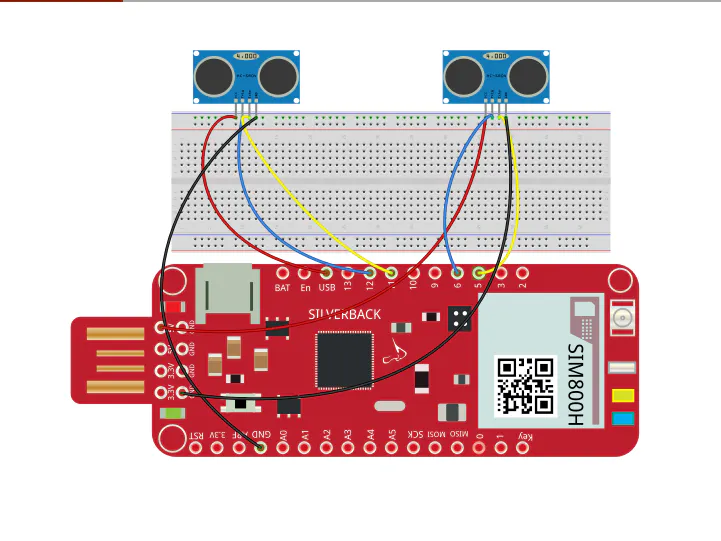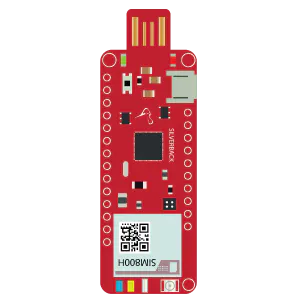# Hand Gesture Control Using Surilli GSM and Python

Controlling few functions of your computer and other applications using hand gestures.

BeginnerFull instructions provided15 minutes263## Things used in this project

### Hardware componentsUltrasonic Sensor - HC-SR04 (Generic)
×2Surilli GSM
×1Jumper wires (generic)
×1×1

### Software apps and online servicesArduino IDE
 Python

## Code

### Hand_Gesture_Control_using_Ultrasonic_Sensor_and_Surilli_GSM

C/C++
```const int trigPin1 = 11; // the number of the trigger output pin ( sensor 1 )
const int echoPin1 = 10; // the number of the echo input pin ( sensor 1 )
const int trigPin2 = 6;  // the number of the trigger output pin ( sensor 2 )
const int echoPin2 = 5;  // the number of the echo input pin ( sensor 2 )

////////////////////////////////// variables used for distance calculation
long duration;
int distance1, distance2;
float r;
unsigned long temp=0;
int temp1=0;
int l=0;
////////////////////////////////

void find_distance (void);

// this function returns the value in cm.
/*we should not trigger the both ultrasonic sensor at the same time.
it might cause error result due to the intraction of the both soundswaves.*/
void find_distance (void)
{
digitalWrite(trigPin1, LOW);
delayMicroseconds(2);
digitalWrite(trigPin1, HIGH);
delayMicroseconds(10);
digitalWrite(trigPin1, LOW);

duration = pulseIn(echoPin1, HIGH, 5000);// here this pulsein function wont wait more then 5000us for the ultrasonic sound to came back. (due to this it wont measure more than 60cm)
// it helps this project to use the gesture control in the defined space.
// so that, it will return zero if distance greater then 60m. ( it helps usually if we remove our hands infront of the sensors ).

r = 3.4 * duration / 2;                  // calculation to get the measurement in cm using the time returned by the pulsein function.
distance1 = r / 100.00;
/////////////////////////////////////////upper part for left sensor and lower part for right sensor
digitalWrite(trigPin2, LOW);
delayMicroseconds(2);
digitalWrite(trigPin2, HIGH);
delayMicroseconds(10);
digitalWrite(trigPin2, LOW);

duration = pulseIn(echoPin2, HIGH, 5000);
r = 3.4 * duration / 2;
distance2 = r / 100.00;
delay(100);
}

void setup()
{
Serial.begin(9600);
pinMode(trigPin1, OUTPUT); // initialize the trigger and echo pins of both the sensor as input and output:
pinMode(echoPin1, INPUT);
pinMode(trigPin2, OUTPUT);
pinMode(echoPin2, INPUT);
delay (1000);

}

void loop()
{
find_distance(); // this function will stores the current distance measured by the ultrasonic sensor in the global variable "distance1 and distance2"
// no matter what, the program has to call this "find_distance" function continuously to get the distance value at all time.

if(distance2<=35 && distance2>=15) // once if we placed our hands in front of the right sensor in the range between 15 to 35cm this condition becomes true.
{
temp=millis();                   // store the current time in the variable temp. (" millis " Returns the number of milliseconds since the Arduino board began running the current program )
while(millis()<=(temp+300))      // this loop measures the distance for another 300 milliseconds. ( it helps to find the difference between the swipe and stay of our hand in front of the right sensor )
find_distance();
if(distance2<=35 && distance2>=15) // this condition will true if we place our hand in front of the right sensor for more then 300 milli seconds.
{
temp=distance2;                         // store the current position of our hand in the variable temp.
while(distance2<=50 || distance2==0)    // this loop will run untill we removes our hand in front of the right sensor.
{
find_distance();                      // call this function continuously to get the live data.
if((temp+6)<distance2)                // this condition becomes true if we moves our hand away from the right sensor (**but in front of it ). here " temp+6 " is for calibration.
{
Serial.println("down");               // send "down" serially.
}
else if((temp-6)>distance2)           // this condition becomes true if we moves our hand closer to the right sensor.
{
Serial.println("up");                // send "up" serially.
}
}
}
else                                     // this condition becomes true, if we only swipe in front of the right sensor .
{
Serial.println("next");                // send "next" serially.
}
}

else if(distance1<=35 && distance1>=15)   // once if we placed our hands in front of the left sensor in the range between 15 to 35cm this condition becomes true.
{

temp=millis();

while(millis()<=(temp+300))
{
find_distance();
if(distance2<=35 && distance2>=15)  // if our hand detects in the right sensor before 300 milli seconds this condition becomes true. ( usually it happens if we swipe our hand from left to right sensor )
{
Serial.println("change");         // send "change" serially.
l=1;                              // store 1 in variable l. ( it avoids the program to enter into the upcoming if condition )
break;                            // break the loop.
}
}

if(l==0)                               // this condition will become true, only if we swipe our hand in front of left sensor.
{
Serial.println("previous");            // send "previous" serially.
while(distance1<=35 && distance1>=15) // this loop will rotate untill we removes our hand infront of the left sensor. this will avoid not to enter this if condition again.
find_distance();
}
l=0;                                  // make l=0 for the next round.
}

}
```

## Credits

### Surilli

196 projects • 47 followers
Surilli is a premiere Internet of Things centric Technology Company aimed at providing cutting edge innovative solutions.